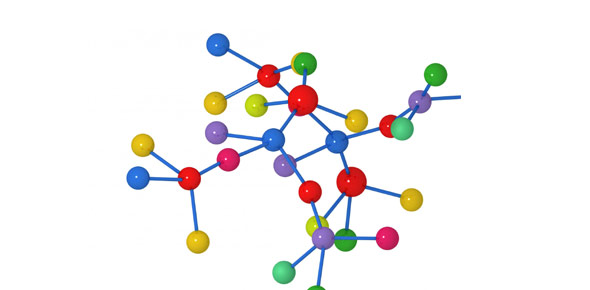# Q1 Interim Review

38 QuestionsSettingsIf you can complete this quiz without using your notes, you should be well prepared for the interim. However, this quiz is NOT complete - there may be material on the interim that is not included on this quiz, so you must continue to review all of your notes.

Related Topics
• 1.
Hydrogen atoms contain one electron.  Two hydrogen atoms can form a bond by sharing their electrons.  Which of these terms identifies the type of bond that forms between two hydrogen atoms as shown in the Bohr diagram below?
• A.

Electronegative

• B.

Covalent

• C.

Polar

• D.

Ionic

• 2.
Ice is ____________ dense than water and is a good ______________ of heat.
• A.

More; conductor

• B.

More; insulator

• C.

Less; conductor

• D.

Less; insulator

• 3.
A water molecule is an example of a ________________ covalent bond.
• A.

Not enough information.

• B.

Non-polar

• C.

Polar

• 4.
What is the most essential element to life?
• A.

Phosphorus

• B.

Calcium

• C.

Oxygen

• D.

Water

• E.

Carbon

• 5.
Which statement about carbon is false?
• A.

It often combines with many other elements.

• B.

It can react with many other elements except for other carbon atoms.

• C.

It is found in both living organisms and some non-living objects.

• 6.
The four elements that make up 96% of living matter are:
• A.

Carbon

• B.

Calcium

• C.

Hydrogen

• D.

Nitrogen

• E.

Oxygen

• F.

Phosphorus

• G.

Zinc

• H.

Iron

• I.

Helium

• 7.
Which type of cell is more complex?
• A.

Prokaryote

• B.

Eukaryote

• 8.
What type of cell is depicted below?
• A.

Plant

• B.

Animal

• C.

Eukaryotic

• D.

Prokaryotic

• 9.
The main difference between prokaryotic and eukaryotic cells is that prokaryotes do not have...
• A.

Cell membranes

• B.

DNA

• C.

Nuclei

• D.

Ribosomes

• 10.
Which of the organelle combinations below can be observed in both plant cells and animal cells?
• A.

Cell wall and chloroplast

• B.

Cell wall and nucleus

• C.

Cell membrane and nucleus

• D.

Chloroplast and nucleus

• 11.
Which is more likely to occur in a plant cell than in an animal cell?
• A.

Creation of protein.

• B.

Diffusion of materials across a membrane.

• C.

Production of glucose.

• D.

Digestion of molecules.

• 12.
What do plants release into the air as a by-product of photosynthesis?
• A.

Oxygen

• B.

Carbon Dioxide

• C.

Sugar

• D.

ATP

• E.

Sugar and oxygen

• 13.
What gas is created as a by-product of cellular respiration?
• A.

Carbon dioxide

• B.

Oxygen

• C.

Oxygen and Water

• D.

ATP

• 14.
What energy-rich molecule is produced by cellular respiration?
• A.

Carbohydrate

• B.

Lipid

• C.

Protein

• D.

Water

• E.

ATP

• F.

Carbon dioxide

• 15.
The process below occurs in the mitochondria of eukaryotic cells.  What is the name of this process?
• A.

Photosynthesis

• B.

Cellular respiration

• C.

Glycolysis

• D.

Calvin Cycle

• 16.
What type of cell is depicted below?  Choose the most specific answer.
• A.

Eukaryote

• B.

Prokaryote

• C.

Plant

• D.

Animal

• 17.
What type of cell is depicted below?  Choose the most specific answer.
• A.

Eukaryote

• B.

Prokaryote

• C.

Plant

• D.

Animal

• 18.
What is the original source of energy in photosynthesis?
• A.

ATP

• B.

Sunlight

• C.

Nucleus

• D.

DNA

• 19.
What is the first stage of cellular respiration?
• 20.
What is the second stage of cellular respiration?
• 21.
What is the third stage of cellular respiration?
• 22.
Which stage(s) is/are anaerobic?
• A.

Glycolysis

• B.

Krebs Cycle

• C.

Electron Transport Chain

• 23.
How many (net) ATP are produced in glycolysis?
• A.

1

• B.

2

• C.

4

• D.

8

• E.

32

• 24.
How many ATP are produced in the Krebs Cycle?
• A.

1

• B.

2

• C.

4

• D.

8

• E.

32

• 25.
How many ATP are produced by the electron transport chain?
• A.

1

• B.

2

• C.

4

• D.

8

• E.

32

• 26.
If glycolysis and the Krebs cycle did not produce electron carriers, how many ATP would cellular respiration produce?(HINT: If there are no electron carriers, which stage of cellular respiration cannot occur?)
• A.

1

• B.

2

• C.

4

• D.

8

• E.

32

• 27.
Where does glycolysis occur?
• 28.
Where does the Krebs cycle occur?
• 29.
Where does the electron transport chain occur?
• 30.
What is the first stage of photosynthesis?
• 31.
What is the second stage of photosynthesis?
• 32.
Where do the light reactions occur?
• 33.
Where do the dark reactions/Calvin cycle occur?
• 34.
What are the inputs of the Calvin cycle?
• A.

Water

• B.

Oxygen

• C.

Carbon Dioxide

• D.

ATP

• E.

Sunlight

• 35.
What are the inputs of the Calvin cycle?
• A.

Water

• B.

Sunlight

• C.

ATP

• D.

Oxygen

• E.

Carbon dioxide

• 36.
What are the outputs of the light reactions?
• A.

Water

• B.

Sunlight

• C.

ATP

• D.

Carbon dioxide

• E.

Oxygen

• 37.
If there were no carbon dioxide, which stage of respiration would be immediately impacted?
• A.

Glycolysis

• B.

Krebs cycle

• C.

Electron Transport Chain

• D.

No impact

• 38.
If there were no sunlight, which stage of photosynthesis would be immediately impacted?
• A.

Light reactions

• B.

Calvin cycle

• C.

No impact